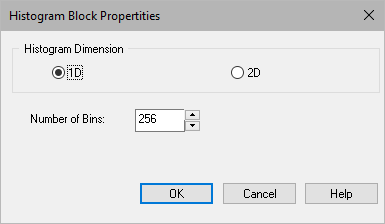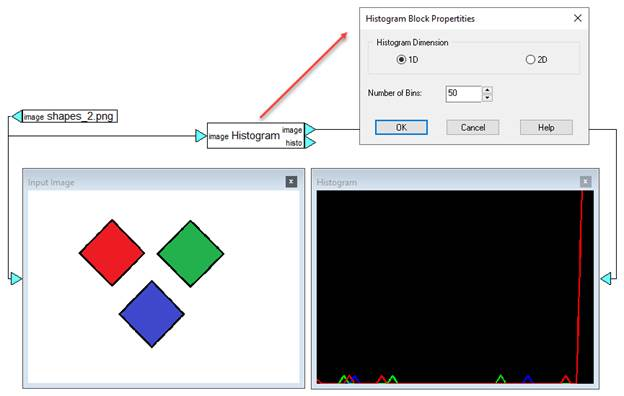### Histogram

Block Category: Analysis

Input Image Requirements: RGB (1D) or HSV (2D)

Description: The Histogram block displays a graphical representation of the distribution of pixel values in an image. It calculates the frequency (y-axis) of pixel values (x-axis) in an image by sorting them into the specified number of bins.#### Histogram Dimension

1D: Splits the image into three channels and calculates the histogram for each channel. It formats the input as an image.

2D: It requires HSV input. It calculates histogram for the H channel. The histogram can be used as input for  the Back Projection block.

Number of Bins: Specifies the number of bins.

#### Example

In this example, a 1D histogram is created with 50 bins to compute the R G B values.